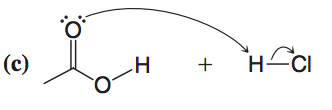Problem: Follow the curved arrows and write the products.

97% (53 ratings)
Problem Details

Follow the curved arrows and write the products.What scientific concept do you need to know in order to solve this problem?

Our tutors have indicated that to solve this problem you will need to apply the Reaction Mechanism concept. You can view video lessons to learn Reaction Mechanism Or if you need more Reaction Mechanism practice, you can also practice Reaction Mechanism practice problems .

What is the difficulty of this problem?

Our tutors rated the difficulty of Follow the curved arrows and write the products. ... as low difficulty.

How long does this problem take to solve?

Our expert Organic tutor, Jonathan took less than a minute to solve this problem. You can follow their steps in the video explanation above.

What professor is this problem relevant for?

Based on our data, we think this problem is relevant for Professor Gallo's class at ULL.

What textbook is this problem found in?

Our data indicates that this problem or a close variation was asked in . You can also practice practice problems .